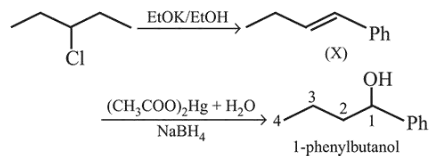# Heating of 2-chloro-1-phenylbutane with EtOK/EtOH gives X as the major product.

Question:

Heating of 2-chloro-1-phenylbutane with EtOK/EtOH gives $X$ as the major product. Reaction of $X$ with $\mathrm{Hg}(\mathrm{OAc})_{2} / \mathrm{H}_{2} \mathrm{O}$ followed by $\mathrm{NaBH}_{4}$ gives $\mathrm{Y}$ as the major product. $Y$ is :

1.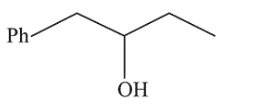2.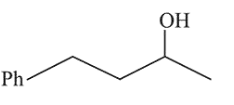3.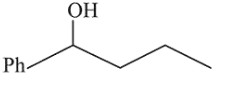4.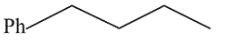Correct Option: , 3

Solution: